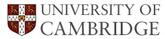Question 1

Substitutional atom diffusion in binary alloys within the cubic crystal system can be described fully with just one diffusion coefficient, the chemical diffusion coefficient (often called the interdiffusion coefficient). Discuss how this situation is modified for the following cases:

1. Non-cubic crystals
2. Ternary alloys
3. Alloys with a very fine grain size
4. Non-ideal solid solutions

1. For non-cubic crystals diffusion is anisotropic (e.g., Se, Zn). Consequently, three principal diffusion coefficients have to be defined for a binary alloy. The flux of solute may not necessarily be parallel to the gradient of solute, except along any one of the three principal axes.
2. For isotropic diffusion in a ternary alloy, a 2 x 2 diffusion matrix has to be defined, since the flux of a particular species also depends on the flux of the other species.
3. In polycrystalline metals, the effective diffusion coefficient is a function of the volume diffusivity and of the grain boundary diffusivity, so that at least two diffusion coefficients have to be known.
4. In non-ideal solutions, the diffusion coefficient is a function of the way in which the chemical potential changes with composition, and is consequently composition dependent.

Question 2

The long-range segregation of aluminium in the intermetallic compound Ni3Al can be minimised by rapidly solidifying liquid droplets of the alloy, in a process known as atomisation. The resulting powder is consolidated to give a fine grained polycrystalline aggregate of Ni3Al. The compound exhibits an increase in strength with temperature, but its creep strength also depends on the rate at which nickel can diffuse through the solid.

Calculate the effective diffusion coefficient of Ni at 1273 K in a NiAl sample with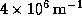of grain boundary area per unit volume, given that the volume diffusivity of Ni in the alloy is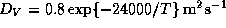and its grain boundary diffusivity is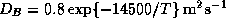.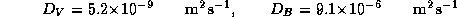The fraction of atoms located at the grain boundaries (i.e.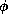), assuming a grain boundary thickness of 1 atom (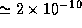m) is given by: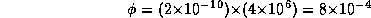The effective diffusivity is thus: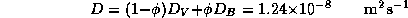Question 3

Discuss the factors controlling the morphology of transformation products during diffusional phase transformations, commenting on both solid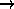liquid and solid-state reactions.

Your answer should include a discussion of interface stability, which to some extent determines particle shape during diffusional growth (whether this is from liquidsolid, solidliquid or solidsolid). However, the shape also depends on the orientation dependence of interface energy and on the minimisation of the strain energy due to any volume change. For displacive transformations the strain energy due to the shape change dominates morphology when the transformations occur under constraint.

Question 4

By considering the conservation of mass at a moving interface, derive an expression for the concentration gradient in the liquid at the solid/liquid interface, during solute diffusion-controlled solidification.

A Pb-0.1Sn wt% alloy is solidified at a rate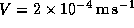. Comment on the shape of the solid-liquid interface during solidification. Assume the following data:

• Temperature gradient in the liquid at the interface =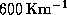.
• Variation in the liquidus temperature of dilute Pb-Sn alloys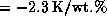Sn
• Distribution coefficient of Sn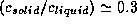.
• Diffusivity of Sn in liquid at solidification temperature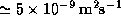.

The diffusion flux of solute away from the moving interface must equal the rate of solute rejection by the precipitate. The diffusion flux is given by Fick's first law, applied at the position of the interface (i.e. at y=0, where y is the co-ordinate with origin at the interface and which is positive in the liquid):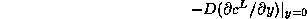where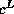is the concentration in the liquid and D the solute diffusivity in the liquid. It follows that for mass to be conserved,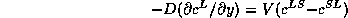where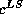and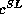are the equilibrium solute concentrations in the liquid and solid respectively, at the interface. If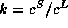,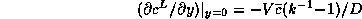and this is the required answer (is the average solute concentration in the alloy and V the interface velocity). Note that the concentration gradient in the liquid at the position of the interface is the term important in determining interface stability, and that it is not necessary to have detailed information about the variation ofas a function of y.

For the second part of the question, we note that constitutional supercooling occurs if the temperature gradient in the liquid (i.e.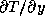) is less than the liquidus temperature gradient at the interface: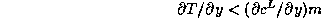where m is the change in liquidus temperature for a unit change in. It follows that for constitutional supercooling,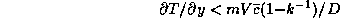and substitution of the various quantities shows that the left hand term isand the right hand term is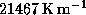. Not only is the liquid constitutionally supercooled, but the extent of the supercooling is large, so that dendritic solidification is to be expected. Whether or not the dendrites are crystallographically faceted depends on how the interface energy varies with interface orientation.

Question 5

Explain the origin of the following phenomena:

1. Shape memory effect;
2. rubber elasticity in metals;
3. transformation induced plasticity (TRIP).

1. The shape memory effect arises because there exists an atomic correspondence between the parent and product phases during martensitic transformation (diffusionless). Consequently, any shape change produced by transformation can be reversed on reverse transformation.
2. During martensitic transformation, several accommodating crystallographic variants of martensite are produced from the parent grains. Each variant represents a large physical deformation (an IPS) on a different system. Under the influence of stress, a martensite variant which is more suited to the stress grows by a diffusionless mechanism while consuming the others, thus causing the specimen to become longer along the direction of the applied stress. Removal of the stress reverses the martensitemartensite transformation and causes the specimen to return to its original form. Thus the metal behaves like a rubber.
3. The formation of martensite can be induced by stress (since the transformation involves a physical deformation). Consequently, if stress concentrations arise in a tensile specimen of a certain kind of an austenitic alloy, they can be relieved by the localised formation of martensite, thus allowing deformation to continue without necking. This is the TRIP phenomenon, whereby necking is delayed, thus increasing the effective plasticity.

Question 6

A 50 at.% Cu-Ni alloy is rapidly cooled and solidifies with a dendrite arm spacing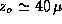m. The alloy is found to be heavily cored, the copper concentration c varying approximately sinusoidally in the z direction across the dendrite arms: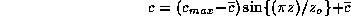whereis the average Cu concentration in the alloy.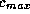is the maximum Cu concentration to be found immediately after casting;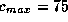at.% immediately after casting.

In order to homogenise the cast structure, the alloy is then annealed at 1200 K. Use Fick's second law to determine the function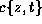, where t is the time at the annealing temperature. Calculate the time needed at 1200 K in order to reduce the maximum copper concentration to 60 at.%, given that the diffusivity of copper at 1200 K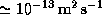.

What would be the effect on the kinetics of homogenisation, of deforming the alloy prior to the annealing treatment?

Discuss the complications that may arise in extending this method to the analysis of homogenisation in ternary alloys and in alloys which do not solidify as a single phase.

Fick's second law states that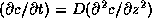. Now,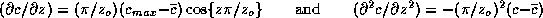This can be integrated to give: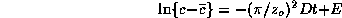where E is an integration constant solved by noting that at t=0,so that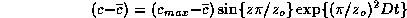The maximum in concentration occurs at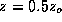; if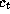is the maximum concentration to be found in the alloy after a time t at the annealing temperature then we have: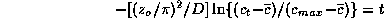so that the time required is 1485 seconds.

Deformation introduces defects and also recrystallisation; both of these provide short-circuit diffusion paths and enhance homogenisation.

For ternary alloys, the simple analysis above fails because the flux of an element does not depend on just its concentration gradient, but also on the gradient of one of the other elements. Cu-Ni alloys show complete miscibility in the solid and liquid phases, so that diffusion is in a single phase. For multiphase systems, chemical potential gradients have to be considered, even when ideal solutions exist in each phase.Examples 7-19 Examples 20-22 Download PDF file